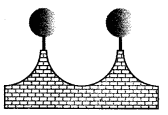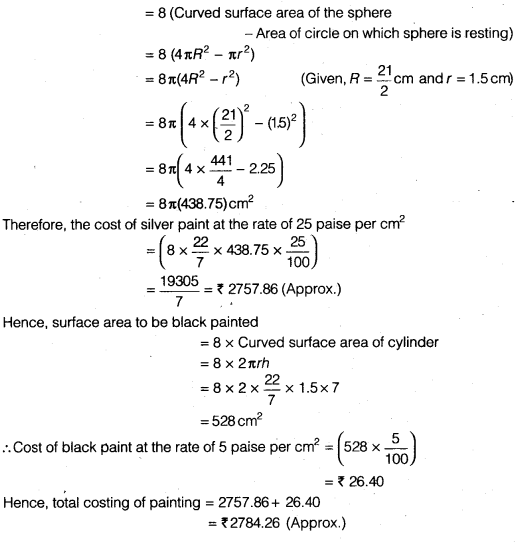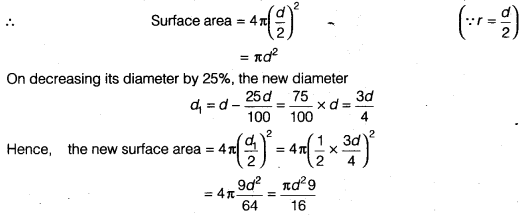NCERT Solutions for Class 9 Maths Chapter 13 Surface Areas and Volumes Ex 13.9 are part of NCERT Solutions for Class 9 Maths. Here we have given NCERT Solutions for Class 9 Maths Chapter 13 Surface Areas and Volumes Ex 13.9.

 Board CBSE Textbook NCERT Class Class 9 Subject Maths Chapter Chapter 13 Chapter Name Surface Areas and Volumes Exercise Ex 13.9 Number of Questions Solved 3 Category NCERT Solutions

## NCERT Solutions for Class 9 Maths Chapter 13 Surface Areas and Volumes Ex 13.9

Question 1.
A wooden bookshelf has external dimensions as follows : Height = 110cm, Depth = 25cm, Breadth = 85cm (see figure). The thickness of the plank is 5 cm everywhere. The external faces are to be polished and the inner faces are to be painted. If the rate of polishing-is 20 paise per cm2 and the rate of pointing is 10 paise per cm2, find the total expenses required for palishing and painting the surface of the bookshelf.Solution:
Here, l = 85 cm b = 25 cm and h = 110 cm
Area of the bookshelf of outer surface = 2 lb + 2bh + hl
= [2(85 x 25)+ 2(110 x 25)+ 85×110] cm2 = (4250 + 5500 + 9350) cm2 = 19100 cm2
Cost of polishing of the outer surface of bookshelf
= 19100 x $$\frac { 20 }{ 100 }$$ = ₹ 3820
Thickness of the plank = 5 cm
Internal height of bookshelf = (110 – 2 x 5) = 100 cm
Internal depth of bookshelf = (25 – 5) = 20 cm
Internal breadth of bookshelf = 85 – 2 x 5 = 75 cm
Hence, area of the internal surface of bookshelf
= 2(75 x 20)+ 2(100x 20)+ 75×100
= 3000 + 4000 + 7500 = 14500 cm2
So, cost of painting of internal surface of bookshelf
= 14500 x $$\frac { 10 }{ 100 }$$ = ₹1450
Hence, total costing of polishing and painting = 3820 + 1450= ₹ 5270

Question 2.
The front compound wall of a house is decorated by wooden spheres of diameter 21 cm, placed on small supports as shown in figure. Eight such spheres are-used for this purpose, and are to be painted silver. Each support is a cylinder of radius 1.5 cm and height 7 cm and is to be painted black. Find the cost of paint required if silver paint costs 25 paise per cm2 and black paint costs 5 paise per cm2.Solution:
It is obvious, we have to subtract the cost of the sphere that is resting on the supports while calculating the cost of silver paint.
Surface area to be silver paintQuestion 3.
The diameter of a sphere is decreased by 25%. By what per cent does its curved surface area decrease?
Solution:
Let d be the diameter of the sphereWe hope the NCERT Solutions for Class 9 Maths Chapter 13 Surface Areas and Volumes Ex 13.9 help you. If you have any query regarding NCERT Solutions for Class 9 Maths Chapter 13 Surface Areas and Volumes Ex 13.9, drop a comment below and we will get back to you at the earliest.